# ICSE Solutions for Chapter 4 Linear Ineqautions Class 10 Mathematics

Question 1: Represent the following inequalities on real number lines:
(i) 2x - 1 < 5
(ii) 3x + 1 ≥ - 5
(iii) 2(2x - 3) ≤ 6
(iv) -4 < x < 4
(v) - 2 ≤ x < 5
(vi) 8 ≥ x > -3
(vii) -5 < x ≤ -1

Solution 1: (i) 2x – 1 < 5
2x < 6
x < 3
Solution on number line is:(ii) 3x +1 ≥ -5
3x  ≥ - 6
x ≥ -2
Solution on number line is:(iii) 2(2x - 3) ≤ 6
2x – 3 ≤ 3
2x ≤ 6
x ≤  3
Solution on number line is: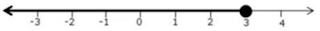(iv) - 4 < x <4
Solution on number line is: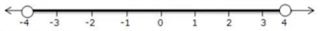(v) -2 ≤ x < 5
Solution is on number line:(vi) 8 ≥ x > - 3
Solution is on number line: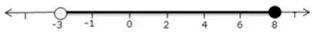(vii) – 5 < x ≤ - 1
Solution is on number line: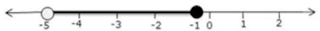Question 2. State, whether the following statements are true or false:
(i) a < b, then a – c < b – c
(ii) If a > b, then a + c > b + C
(iii) If a < b, then ac > bc
(iv) If a > b, then a/c < b/c
(v) If a – c > b - d, then a + d > b + c
(vi) If a < b, and c > 0, then a – c > b - c
Where a, b, c and d are real numbers and c ≠ 0.

Solution 2:
(i) a < b  ⇒ a – c < b - c
The given statement is true.

(ii) If a > b ⇒ a + c > b + c
The given statement is true.

(iii) If a < b ⇒ ac < bc
The given statement is false.

(iv) If a > b ⇒ a/c > b/c
The given statement is false.

(v) If a – c > b – d ⇒ a + d > b + c
The given statement is true.

(vi) If a < b ⇒ a – c < b - c (Since, c > 0)
The given statement is false.

Question 3. If x € N, find the solution set of inequations.
(i) 5x + 3 ≤ 2x + 18
(ii) 3x - 2 < 19 - 4x

Solution 3:
(i) 5x + 3 ≤ 2x + 18
5x - 2x ≤ 18 - 3
3x ≤ 15
x ≤ 5
Since, x ∊ N, therefore solution set is {1,2,3,4,5}.

(ii) 3x - 2 < 19 - 4x
3x + 4x < 19 + 2
7x < 21
x < 3
Since, x ∊ N, therefore solution set is {1, 2}.

Question 4: If the replacement set is the set of whole numbers, solve:
(i) x + 7 ≤ 11
(ii) 3x - 1 > 8
(iii) 8 – x > 5
(iv) 7- 3x  ≥ -1/2
(v) x – 3/2 < 3/2 - x
(vi) 18 ≤ 3x - 2

Solution 4:
(i) x + 7 ≤ 11
x ≤ 11 – 7
x ≤ 4
Since, the replacement set = W (set of whole numbers)
⇒ Solution set = {0, 1, 2, 3,4}

(ii) 3x – 1 > 8
3x > 8 + 1
x > 3
Since, the replacement set = W (set of whole numbers)
⇒ Solution set = {4, 5, 6, ...}

(iii) 8 – x > 5
- x > 5 - 8
- x > -3
x < 3
Since, the replacement set = W (set of whole numbers)
⇒ Solution set = {0,1,2)

(iv) 7 – 3x ≥ -1/2
-3x ≥ -1/2 - 7
-3x ≥ - 15/2
x ≤ 5/2
Since, the replacement set = W (set of whole numbers)
∴ Solution set = {0, 1, 2}

(v) x – 3/2 < 3/2 – x
x + x < 3/2 + 3/2
2x < 3
x < 3/2
Since, the replacement set = W(set of whole numbers)
∴ Solution set = {0, 1}

(vi) 18 ≤ 3x – 2
18 + 2 ≤ 3x
20 ≤ 3x
x ≥ 20/3
Since, the replacement set = W (set of whole numbers)
∴ Solution set = {7, 8, 9…….}

Question 5: X ∊ (real numbers) and -1 < 3 - 2x ≤ 7, evaluate x and represent it on a number line.

Solution 5:
-1 < 3 - 2x ≤ 7
-1 < 3 - 2x and 3 - 2x  ≤  7
2x < 4 and -2x ≤ 4
x < 2 and x ≥ -2
Solution set = {-2 ≤ x < 2, x € R}
Thus, the solution can be represented on a number line as: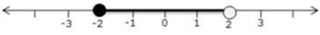Question 6: List the elements of the solution set of the inequation
-3 < x -2 ≤ 9 – 2x; x ∊ N.

Solution 6: -3 < x – 2 ≤ 9 - 2x
-3 < x - 2 and x – 2 ≤ 9 - 2x
-1 < x and 3x ≤ 11
-1 < x ≤ 11/3
Since,  x ∊ N
∴ Solution set = {1,2,3}

Question 7: Find the range of values of x which satisfies
-2.2/3 ≤ x + 1/3 < 3.1/3; x € R.
Graph these values of x on the number line.

Solution 7:
-2.2/3 ≤  x + 1/3 and x + 1/3 < 3.1/3
⇒  - 8/3 ≤ x + 1/3 and x + 1/3 < 10/3
⇒ - 8/3 – 1/3 ≤ x and x < 10/3 – 1/3
⇒  - 9/3 ≤ x and x < 9/3
⇒  -3 ≤ x and x < 3
∴ 3 ≤ x < 3
The required graph of the solution set is: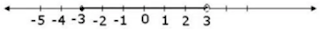Question 8. Find the values of x, which satisfy the inequation:
-2 ≤ ½ - 2x/3 < 1.5/6; x € N.
Graph the solution on the number line.

Solution 8:
-2 ≤ ½ -  2x/3 < 1.5/6
-2 ≤ ½ - 2x/3  and ½  - 2x/3 < 1.5/6
-5/2 ≤ - 2x/3  and  - 2x/3 < 8/6
15/4 ≥ x   and x > -2
3.75 ≥ x  and x > - 2
Since, x € N
∴ Solution set = {1,2,3}
The required graph of the solution set is: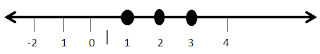Question 9. Given x € (real numbers), find the range of values of x for which -5 ≤ 2x – 3 < x + 2 and represent it on a number line.

Solution 9: -5 ≤ 2x – 3 < x + 2
-5 ≤ 2x - 3 and 2x – 3 < x + 2
-2 ≤ 2x and x < 5
-1 ≤ x and x < 5
Required range is -1 ≤ x < 5.
The required graph is: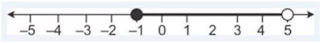Question 10: If 5x – 3 ≤ 5 + 3x ≤ 4x + 2, express it as a ≤ x ≤ b and then state the values of a and b.

Solution 10: 5x – 3 ≤ 5 + 3x ≤ 4x + 2
5x – 3 ≤ 5 + 3x and 5 + 3x ≤ 4x + 2
2x ≤ 8 and –x ≤  -3
x ≤ 4 and x ≥ 3
Thus, 3 ≤ x ≤ 4
Hence, a = 3 and b = 4.